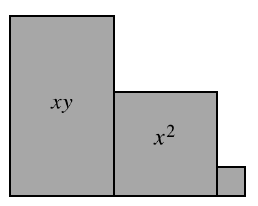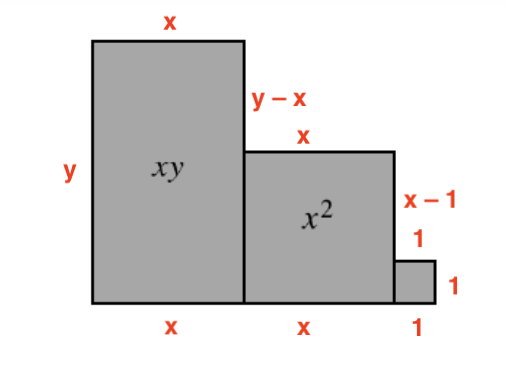### Home > CC3MN > Chapter 3 > Lesson 3.1.6 > Problem3-59

3-59.Write an expression that represents the perimeter of the shape built with algebra tiles at right. Then find the perimeter if $x=3$ units and $y=7$ units.

Find the length of each side, and then sum the lengths.Once you find an expression for the perimeter, substitute $3$ for $x$ and $7$ for $y$.

$28 \; \text{units}$